### LINCON Statement

LINCON constraint <, constraint …> ;

where constraint represents one of the following:number operator linear-termlinear-term operator number and linear-term is <+|-> <coefficient *> parameter <<+|-> <coefficient *> parameter …>

The LINCON statement specifies a set of linear equality or inequality constraints of the following form: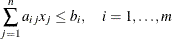The constraints must be separated by commas. Each linear constraint i in the statement consists of a linear combination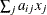of a subset of the n parameters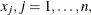and a constant value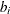separated by a comparison operator. Valid operators are <=, <, >=, >, and = or, equivalently, LE, LT, GE, GT, and EQ. PROC CALIS cannot enforce the strict inequalities < or >. Note that the coefficients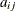in the linear combination must be constant numbers and must be followed by an asterisk and the name of a parameter (that is, listed in the PARMS, main, or subsidiary model specification statements). The following is an example of the LINCON statement that sets a linear constraint on parameters x1 and x2:

lincon       x1 + 3 * x2 <= 1;


Although you can express boundary constraints of individual parameters in LINCON statements, it is much more convenient to specify these boundary constraints with the BOUNDS statement. For example, the following LINCON statement essentially specifies boundary constraints on four parameters:

lincon       x1 <= 1,
x2 <= 1,
v1 > 0,
v2 > 0;


Instead of using the LINCON statement, you can specify the following BOUNDS statement:

bounds       x1 x2 <= 1,
v1 v2 >  0;


Another advantage of using the BOUNDS statement for setting bounds for individual parameters is that when a boundary constraint becomes active in the solution (that is, when the final estimate is on the boundary), a Lagrange multiplier test on releasing the boundary constraint would be conducted when you also specify the MOD option. However, no Lagrange multiplier test would be conducted if you specified the boundary constraint with the LINCON statement.